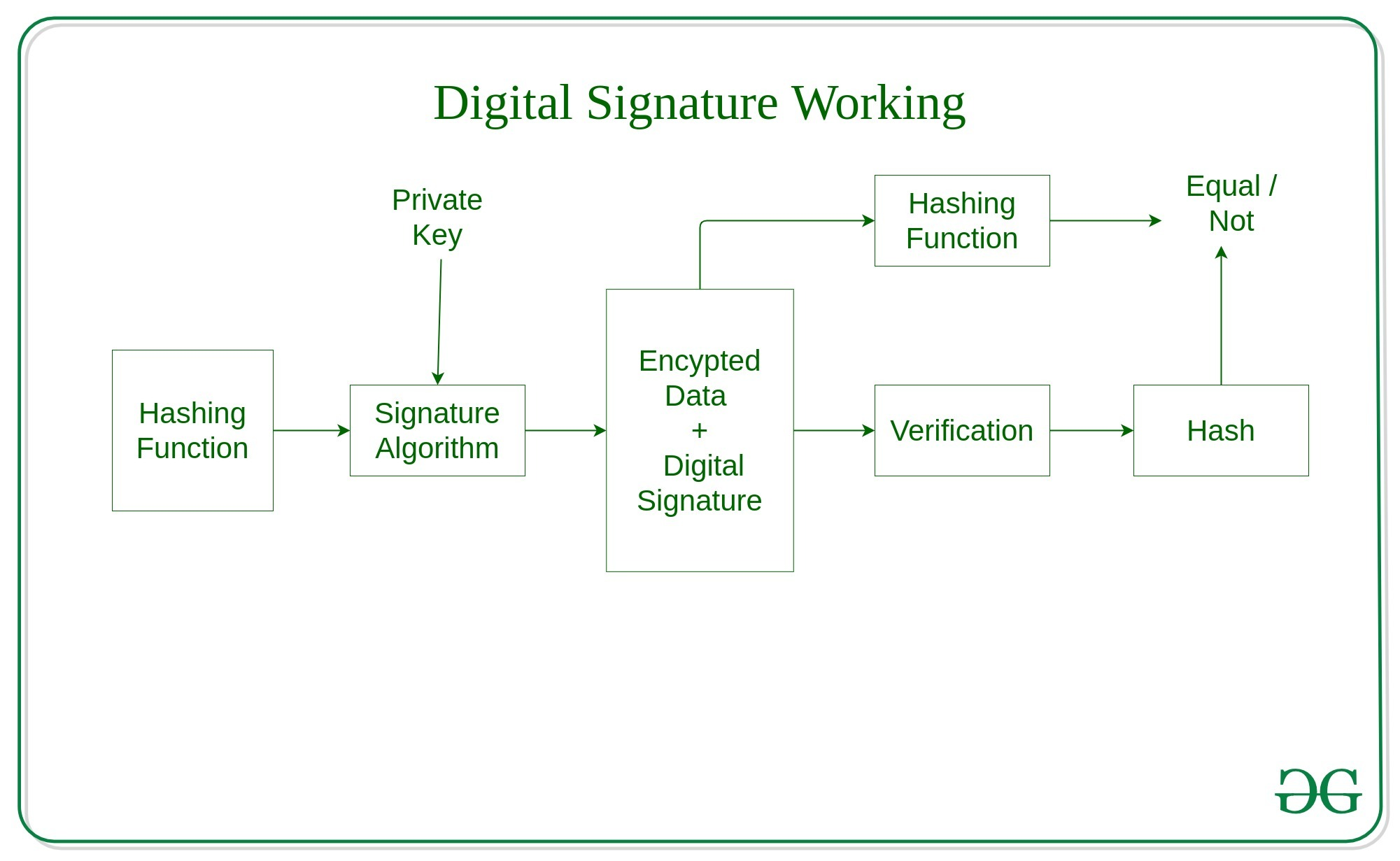GeeksforGeeks App
Open AppBrowser
Continue

# Java implementation of Digital Signatures in Cryptography

Digital Signatures are an Asymmetrically encrypted hash of a digital message(data). It is a value that can provide a guarantee of authenticity, non-repudiation, and integrity. In other terms, it means you can verify the sender, date & time and message content have not been revealed or compromised.

Note: You can refer this link for better understanding of cryptographic terms.

Calculation of Digital Signature

Digital Signatures are often calculated using elliptical curve cryptography, especially in IoT devices, but we will be using RSA for demonstration purposes. First, we will take the input message and create a hash of it using SHA-256 because of its speed and security, and we will then encrypt that hash with the private key from Asymmetric key pair. On the other side, the receiver will decrypt it using the public key and compare the hash to ensure they are indeed the same.Digital Signature Flow

• Let “A” and “B” be the fictional actors in the cryptography system for better understanding.
• “A” is the sender and calculates the hash of the message and attaches signature which he wants to send using his private key.
• The other side “B” hashes the message and then decrypts the signature with A’s public key and compares the two hashes
• If “B” finds the hashes matching then the message has not been altered or compromised.

### Implementing Digital Signatures

Let us implement the digital signature using algorithms SHA and RSA and also verify if the hash matches with a public key.

Approach:

• Create a method named Create_Digital_Signature() to implement Digital Signature by passing two parameters input message and the private key. In this method we will get an instance of the signature object passing the signing algorithm and assign it with a private key and finally pass the input this will return byte array.
```public static byte[] Create_Digital_Signature(byte[] input, PrivateKey privateKey);
signature.initSign(privateKey);
signature.update(input);
```
• The next step is to generate asymmetric key pair using RSA algorithm and SecureRandom class functions.
```SecureRandom secureRandom =new SecureRandom();
KeyPairGenerator keyPairGenerator = KeyPairGenerator.getInstance(ALGORITHM);
```
• Finally verifying the signature using public key. Verify_Digital_Signature() method is used to check whether the signature matches by passing it the input, signature, and public key.
```Signature signature = Signature.getInstance(SIGNING_ALGORITHM);
signature.initVerify(publickey);
signature.update(input);```

Example:

Input:msg = “GEEKSFORGEEEKS IS A COMPUTER SCIENCE PORTAL”
Output:
Signature Value:
80429D3FA203437B4098CAF774D96C827B6CC2489F437A82926DA2EFCE64EF68FB33235B9F6BA8E3B033235B9F6BA8
Verification: true

Below is the implementation:

 `// Java implementation for Generating``// and verifying the digital signature`` ` `package` `java_cryptography;`` ` `// Imports``import` `java.security.KeyPair;``import` `java.security.KeyPairGenerator;``import` `java.security.PrivateKey;``import` `java.security.PublicKey;``import` `java.security.SecureRandom;``import` `java.security.Signature;``import` `java.util.Scanner;`` ` `import` `javax.xml.bind.DatatypeConverter;`` ` `public` `class` `Digital_Signature_GeeksforGeeks {`` ` `    ``// Signing Algorithm``    ``private` `static` `final` `String``        ``SIGNING_ALGORITHM``        ``= ``"SHA256withRSA"``;``    ``private` `static` `final` `String RSA = ``"RSA"``;``    ``private` `static` `Scanner sc;`` ` `    ``// Function to implement Digital signature``    ``// using SHA256 and RSA algorithm``    ``// by passing private key.``    ``public` `static` `byte``[] Create_Digital_Signature(``        ``byte``[] input,``        ``PrivateKey Key)``        ``throws` `Exception``    ``{``        ``Signature signature``            ``= Signature.getInstance(``                ``SIGNING_ALGORITHM);``        ``signature.initSign(Key);``        ``signature.update(input);``        ``return` `signature.sign();``    ``}`` ` `    ``// Generating the asymmetric key pair``    ``// using SecureRandom class``    ``// functions and RSA algorithm.``    ``public` `static` `KeyPair Generate_RSA_KeyPair()``        ``throws` `Exception``    ``{``        ``SecureRandom secureRandom``            ``= ``new` `SecureRandom();``        ``KeyPairGenerator keyPairGenerator``            ``= KeyPairGenerator``                  ``.getInstance(RSA);``        ``keyPairGenerator``            ``.initialize(``                ``2048``, secureRandom);``        ``return` `keyPairGenerator``            ``.generateKeyPair();``    ``}`` ` `    ``// Function for Verification of the``    ``// digital signature by using the public key``    ``public` `static` `boolean``    ``Verify_Digital_Signature(``        ``byte``[] input,``        ``byte``[] signatureToVerify,``        ``PublicKey key)``        ``throws` `Exception``    ``{``        ``Signature signature``            ``= Signature.getInstance(``                ``SIGNING_ALGORITHM);``        ``signature.initVerify(key);``        ``signature.update(input);``        ``return` `signature``            ``.verify(signatureToVerify);``    ``}`` ` `    ``// Driver Code``    ``public` `static` `void` `main(String args[])``        ``throws` `Exception``    ``{`` ` `        ``String input``            ``= ``"GEEKSFORGEEKS IS A"``              ``+ ``" COMPUTER SCIENCE PORTAL"``;``        ``KeyPair keyPair``            ``= Generate_RSA_KeyPair();`` ` `        ``// Function Call``        ``byte``[] signature``            ``= Create_Digital_Signature(``                ``input.getBytes(),``                ``keyPair.getPrivate());`` ` `        ``System.out.println(``            ``"Signature Value:\n "``            ``+ DatatypeConverter``                  ``.printHexBinary(signature));`` ` `        ``System.out.println(``            ``"Verification: "``            ``+ Verify_Digital_Signature(``                  ``input.getBytes(),``                  ``signature, keyPair.getPublic()));``    ``}``}`

My Personal Notes arrow_drop_up